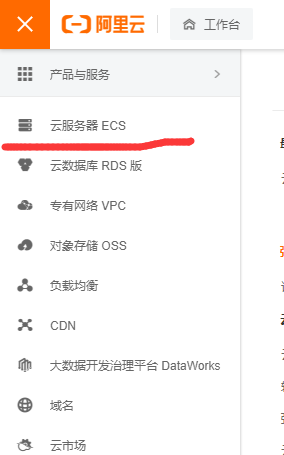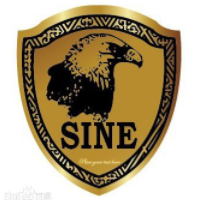[LeetCode] Best Time to Buy and Sell Stock III

+关注继续查看

Say you have an array for which the ith element is the price of a given stock on day i.

Design an algorithm to find the maximum profit. You may complete at most two transactions.

Note:
You may not engage in multiple transactions at the same time (ie, you must sell the stock before you buy again).

实现代码

/*****************************************************************
*  @Author   : 楚兴
*  @Date     : 2015/2/22 18:08
*  @Status   : Accepted
*  @Runtime  : 16 ms
******************************************************************/
#include <iostream>
#include <vector>
#include <algorithm>

using namespace std;

class Solution {
public:
int maxProfit(vector<int> &prices) {
int len = prices.size();
if (len == 0)
{
return 0;
}
vector<int> left(len, 0);
vector<int> right(len, 0);

int i = 0;
int low = prices;
int profit = 0;
while (i < len - 1)
{
if (prices[i] < low)
{
low = prices[i];
}
else if (prices[i] >= prices[i + 1])
{
profit = max(profit, prices[i] - low);
}
left[i] = profit;
i++;
}
profit = max(profit, prices[i] - low);
left[i] = profit;

int high = prices[i];
profit = 0;
while(i > 0)
{
if (prices[i] > high)
{
high = prices[i];
}
else if (prices[i] <= prices[i - 1])
{
profit = max(profit, high - prices[i]);
}
right[i] = profit;
i--;
}
profit = max(profit, high - prices[i]);
right[i] = profit;

i = 0;
int max_profit = 0;
while (i < len)
{
max_profit = max(max_profit, left[i] + right[i]);
i++;
}

return max_profit;
}
};

int main()
{
int num[] = {1,2,3,4,5,6};
vector<int> n(num, num + sizeof(num)/sizeof(int));

Solution s;
int profit = s.maxProfit(n);
cout<<profit<<endl;
}10056 06759 014478 02507 013869 0windows server 2008阿里云ECS服务器安全设置

9156 07359 054746 04497 022377 0

344

0

《2021云上架构与运维峰会演讲合集》

《零基础CSS入门教程》

《零基础HTML入门教程》